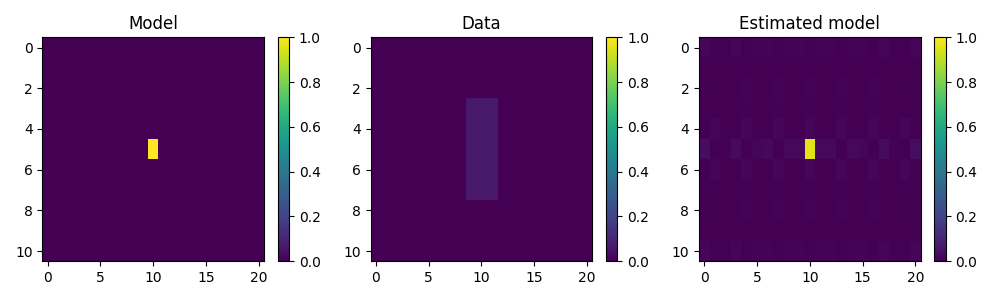# 2D Smoothing#

This example shows how to use the pylops.Smoothing2D operator to smooth a multi-dimensional input signal along two given axes.

import matplotlib.pyplot as plt
import numpy as np

import pylops

plt.close("all")


Define the input parameters: number of samples of input signal (N and M) and lenght of the smoothing filter regression coefficients ($$n_{smooth,1}$$ and $$n_{smooth,2}$$). In this first case the input signal is one at the center and zero elsewhere.

N, M = 11, 21
nsmooth1, nsmooth2 = 5, 3
A = np.zeros((N, M))
A[5, 10] = 1

Sop = pylops.Smoothing2D(nsmooth=[nsmooth1, nsmooth2], dims=[N, M], dtype="float64")
B = Sop * A


After applying smoothing, we will also try to invert it.

Aest = (Sop / B.ravel()).reshape(Sop.dims)

fig, axs = plt.subplots(1, 3, figsize=(10, 3))
im = axs.imshow(A, interpolation="nearest", vmin=0, vmax=1)
axs.axis("tight")
axs.set_title("Model")
plt.colorbar(im, ax=axs)
im = axs.imshow(B, interpolation="nearest", vmin=0, vmax=1)
axs.axis("tight")
axs.set_title("Data")
plt.colorbar(im, ax=axs)
im = axs.imshow(Aest, interpolation="nearest", vmin=0, vmax=1)
axs.axis("tight")
axs.set_title("Estimated model")
plt.colorbar(im, ax=axs)
plt.tight_layout()Total running time of the script: ( 0 minutes 0.553 seconds)

Gallery generated by Sphinx-Gallery